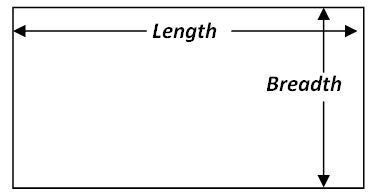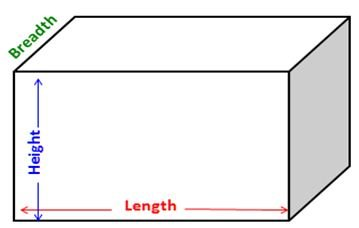# Definition Of Length

## Definition Of Length

Length is the term used for representing the size of an object /distance/ from one end to another end.

It is the greater measurement for all two dimensional figures (figures for which only length and breadth are given).
Example: For every rectangle length is larger than the breadth.Length is the highest measurement for all three dimensional figures (Figures for which Length, Breadth, and Height are given).
Example: For a cuboids� the length is the highest measurement.Generally length is measured in two types of different units.
1. Customary system 2. Metric system
In metric system units for length are millimeters, centimeters, meters, Kilometers.
1 Kilometer = 1000 meters
1 Meter = 100 centimeters
1 centimeter = 10 millimeters.
In customary system (Specially followed in America) units for length are Inch, foot, yard, and mile.
1 Yard = 3 feet
1 Foot = 12 inches

#### Worked Example

How many centimeters are equal to 2 Km?

A. 200
B. 2000
C. 20 000
D. 20 0000